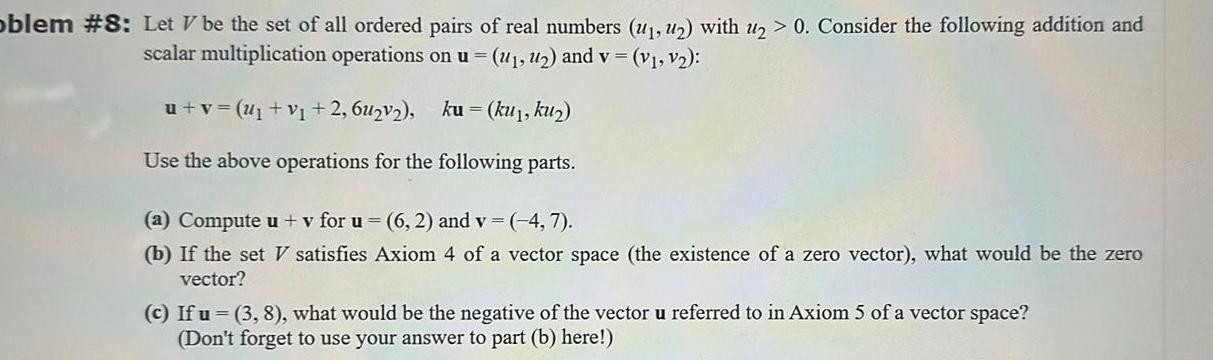Question:

# oblem 8 Let V be the set of all ordered pairs of real

Last updated: 11/15/2023oblem 8 Let V be the set of all ordered pairs of real numbers with u 0 Consider the following addition and scalar multiplication operations on u and v v v u v U v 2 6u v ku ku1 ku Use the above operations for the following parts a Compute u v for u 6 2 and v 4 7 b If the set V satisfies Axiom 4 of a vector space the existence of a zero vector what would be the zero vector c If u 3 8 what would be the negative of the vector u referred to in Axiom 5 of a vector space Don t forget to use your answer to part b here